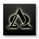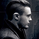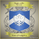Dilihat 32064 kali
Hello, Murrey Math lovers, Thanks for those who showed interest on this. Based on a request, I have updated the plot / candle coloring, for Version - 2.

This has been in the queue for a while.

There was a Glitch found with the Multiplier. Will Fix in the next version. The Current Version (and the previous version) only supports 1/8 fractions. Will not support 0.25. The code needs to be updated, to automate the fractal line glitches for other ratios, Planned for future update.

Good Luck and Enjoy the Colorful Oscillator. Please keep your suggestions flowing. Lets make it better.
```// Created & Developed by Ucsgears, based on Murrey Math Line Principle
// Version 2 - March, 12 - 2015

study(title="UCS_Murrey's Math Oscillator", shorttitle="UCS_MMLO", overlay=false, precision = 2)

// Inputs
length = input(100, minval = 10, title = "Look back Length")
mult = input(0.125, title = "Mutiplier; Only Supports 0.125 = 1/8")
lines = input(true, title= "Show Murrey Math Fractals")
bc = input(true, title = "Show Bar Colors Based On Oscillator")

// Donchanin Channel
hi = highest(high, length)
lo = lowest(low, length)
range = hi - lo
multiplier = (range) * mult
midline = lo + multiplier * 4

oscillator = (close - midline)/(range/2)

a = oscillator > 0 and oscillator < mult*2
b = oscillator > 0 and oscillator < mult*4
c = oscillator > 0 and oscillator < mult*6
d = oscillator > 0 and oscillator < mult*8

z = oscillator < 0 and oscillator > -mult*2
y = oscillator < 0 and oscillator > -mult*4
x = oscillator < 0 and oscillator > -mult*6
w = oscillator < 0 and oscillator > -mult*8

colordef = a ? #ADFF2F : b ? #32CD32 : c ? #3CB371 : d ? #008000 : z ? #CD5C5C : y ? #FA8072 : x ? #FFA07A : w ? #FF0000 : blue

plot (oscillator, color = colordef, title = "Murrey Math Oscillator", style = columns, transp = 60)
plot(0, title = "Zero Line", color = gray, linewidth = 4)

plot(lines == 1 ? mult*2 : na, title = "First Positive Quadrant", color = gray, linewidth = 1)
plot(lines == 1 ? mult*4 : na, title = "Second Positive Quadrant", color = gray, linewidth = 1)
p3 = plot(lines == 1 ? mult*6 : na, title = "Third Positive Quadrant", color = gray, linewidth = 2)
p4 = plot(lines == 1 ? mult*8 : na, title = "Fourth Positive Quadrant", color = gray, linewidth = 1)
plot(lines == 1 ? -mult*2 : na, title = "First Negative Quadrant", color = gray, linewidth = 1)
plot(lines == 1 ? -mult*4 : na, title = "Second Negative Quadrant", color = gray, linewidth = 1)
p2 = plot(lines == 1 ? -mult*6 : na, title = "Third Negative Quadrant", color = gray, linewidth = 2)
p1 = plot(lines == 1 ? -mult*8 : na, title = "Fourth Negative Quadrant", color = gray, linewidth = 1)

fill (p1,p2, color = orange)
fill (p3,p4, color = lime)

// Bar Color Oversold and Overbought
bcolor = bc == 1 ? colordef : na
barcolor(bcolor)```

## KomenI think this is a fantastic piece of work. I hope to see how to best combine it with some of your other excellent scripts. It will take a few days for me to find the time to give it the kind of testing it deserves. If you have any suggestions on the best candidates for the combination feel free to suggest them as I will be looking at a lot of combinations.
BalasThnx for the great work on this indicator. I've one question. How should I interpret price going up or down but the bar color indicating the opposite colorwise, e.g. price goes up but the inside of the bar has a red color (even though it surpassed previous price level where the bar color was green)?
BalasGood afternoon! Thanks for the great indicator. Tell me, what does purple bar mean? Thank you!
BalasAWESOME ..... ucsGEARS It's just beautiful ...& comes very close to the Holy Grail or Silver Bullet of Trading ! Thank You SO Much !
Balas